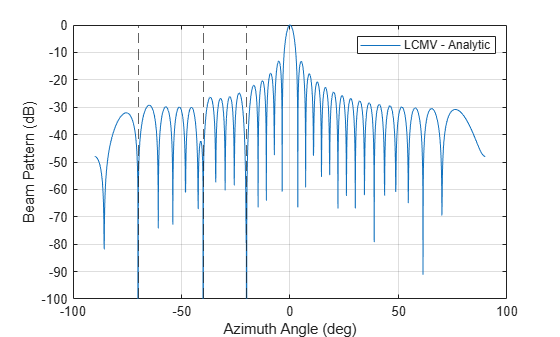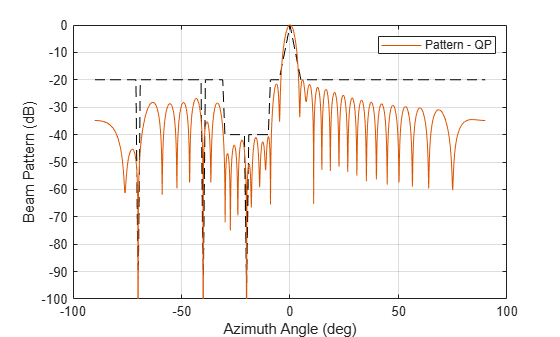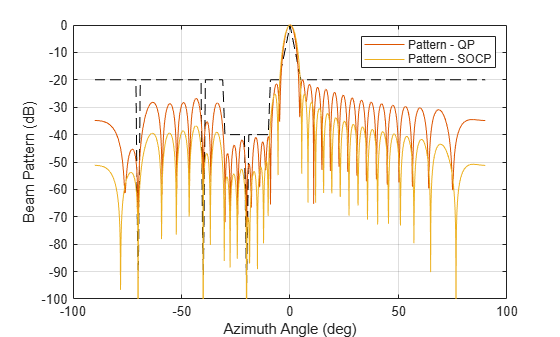# Array Pattern Synthesis Part II: Optimization

This example shows how to use optimization techniques to perform pattern synthesis. Using minimum variance beamforming as an illustration, this example covers how to set up the pattern synthesis as an optimization problem and then solve it using optimization solvers.

Pattern synthesis can be achieved using a variety of techniques. The Array Pattern Synthesis Part I: Nulling, Windowing, and Thinning example introduced nulling and windowing techniques.

This example requires Optimization Toolbox™.

### Minimum Variance Beamforming

Compared to a single element, a major benefit of a phased array is the capability of forming beams. In general, an N-element phased array provides N degrees of freedom when synthesizing a pattern, meaning that one can adjust N weights, one for each element, to control the shape of the beam to satisfy some pre-defined constraints.

Many popular beamforming techniques can be expressed as a optimization problem. For example, the popular minimum variance distortionless response (MVDR) beamformer is used to minimize the total noise output while preserving the signal from a given direction. Mathematically, the MVDR beamforming weights can be found by solving the following optimization problem

`$\begin{array}{l}{\mathit{w}}_{\mathit{o}}=\text{\hspace{0.17em}}{\mathrm{min}}_{\mathit{w}\text{\hspace{0.17em}}}{\mathit{w}}^{\mathit{H}}\mathit{R}\text{\hspace{0.17em}}\mathit{w}\\ \text{\hspace{0.17em}\hspace{0.17em}\hspace{0.17em}\hspace{0.17em}\hspace{0.17em}\hspace{0.17em}\hspace{0.17em}\hspace{0.17em}\hspace{0.17em}\hspace{0.17em}\hspace{0.17em}\hspace{0.17em}}\mathrm{s}.\mathrm{t}.\mathit{B}\left({\theta }_{0},\mathit{w}\right)=1\end{array}$`

where $\mathit{R}$ is the covariance matrix, $\mathit{B}\left({\theta }_{0},\mathit{w}\right)$ is the beam response towards the direction ${\theta }_{0}$ with the weights vector $\mathit{w}$, and ${\mathit{w}}_{\mathit{o}}$ is the vector containing the optimal weights. Because the beam pattern can be written as

`$\mathit{B}\left(\theta \text{\hspace{0.17em}},\mathit{w}\right)={\mathit{w}}^{\mathit{H}}\mathit{v}\left(\theta \text{\hspace{0.17em}}\right)$`

where $\mathit{v}\left(\theta \right)$ is the steering vector corresponding to the angle $\theta \text{\hspace{0.17em}}$, the constraints have a linear relation in the complex domain.

The distortionless constraint at ${\theta }_{0}$ only takes 1 degree of freedom in the design space. Since an N-element array can handle N-1 constraints, the MVDR beamformer can be extended to include more constraints, which results in a linear constraints minimum variance (LCMV) beamformer. The extra constraints in an LCMV beamformer are often used to null out interferences from given directions. Therefore, such an LCMV beamformer is a solution to the following optimization problem

`$\begin{array}{l}{\mathit{w}}_{\mathit{o}}=\text{\hspace{0.17em}}{\mathrm{min}}_{\mathit{w}\text{\hspace{0.17em}}}{\mathit{w}}^{\mathit{H}}\mathit{R}\text{\hspace{0.17em}}\mathit{w}\\ \text{\hspace{0.17em}\hspace{0.17em}\hspace{0.17em}\hspace{0.17em}\hspace{0.17em}\hspace{0.17em}\hspace{0.17em}\hspace{0.17em}\hspace{0.17em}\hspace{0.17em}\hspace{0.17em}\hspace{0.17em}}\mathrm{s}.\mathrm{t}.\mathit{B}\left({\theta }_{0},\mathit{w}\right)=1\\ \text{\hspace{0.17em}\hspace{0.17em}\hspace{0.17em}\hspace{0.17em}\hspace{0.17em}\hspace{0.17em}\hspace{0.17em}\hspace{0.17em}\hspace{0.17em}\hspace{0.17em}\hspace{0.17em}\hspace{0.17em}\hspace{0.17em}\hspace{0.17em}\hspace{0.17em}\hspace{0.17em}\hspace{0.17em}\hspace{0.17em}\hspace{0.17em}\hspace{0.17em}}\mathit{B}\left({\theta }_{\mathit{k}},\mathit{w}\right)=0\text{\hspace{0.17em}\hspace{0.17em}\hspace{0.17em}\hspace{0.17em}\hspace{0.17em}\hspace{0.17em}}\mathit{k}=1,2,\dots ,\mathit{K}\end{array}$`

where ${\theta }_{\mathit{k}}$ represents the $\mathit{k}$th nulling direction and there are $\mathit{K}$ nulling directions in total. Because all constraints are linear in the complex domain, the LCMV beamforing weights can be derived analytically using the Lagrange multiplier method.

Next, a 32-element ULA with half wavelength spacing is used to demonstrate how to perform array synthesis using the LCMV approach. Note that even though this example uses a linear array, the technique applies to any array geometry. Assume that the signal of interest is at 0 degrees azimuth and the interferences are at -70, -40, and -20 degrees azimuth. The noise power is assumed to be at 40 dB below signal. The closed form solution for LCMV weights can be computed using the `lcmvweights` function.

```N = 32; pos = (0:N-1)*0.5; % position of elements ang_i = [-70 -40 -20]; % interference angles ang_d = 0; % desired angle Rn = sensorcov(pos,ang_i,db2pow(-40)); % Noise covariance matrix sv_c = steervec(pos,[ang_d ang_i]); % Linear constraints r_c = [1 zeros(size(ang_i))]'; % Desired response w_lcmv = lcmvweights(sv_c,r_c,Rn); % LCMV weights ang_plot = -90:0.1:90; sv_plot = steervec(pos,ang_plot); plcmv = plot(ang_plot,mag2db(abs(w_lcmv'*sv_plot)),'b'); hold on; plot([ang_i;ang_i],repmat([-100;0],1,size(ang_i,2)),'k--','LineWidth',1.5); hold off; ylim([-100 0]); grid on; legend(plcmv,'LCMV - analytical'); xlabel('Azimuth Angle (deg)'); ylabel('Beam Pattern (dB)');```The figure shows that the computed weights satisfy all constraints.

### LCMV Beamforming Using Quadratic Programming

Since LCMV beamforming can be described as an optimization problem, it would be useful to see how the problem can be solved using optimization techniques. When a closed form solution is not available, for example when constraints include inequalities, such workflow becomes important.

When using optimization techniques to perform pattern synthesis tasks, the first step is to pick a solver. Since solvers are often developed to address a particular problem formulation, it is of utmost importance to make the right choice.

The quadratic programming (QP) solver is often used to solve a quadratic objective function with linear constraints. The formulation is given by

`$\begin{array}{l}{\mathit{w}}_{\mathit{o}}=\text{\hspace{0.17em}}{\mathrm{min}}_{\mathit{w}\text{\hspace{0.17em}}}\frac{1}{2}{\mathit{w}}^{\mathit{T}}\mathit{H}\text{\hspace{0.17em}}\mathit{w}+{\mathit{f}}^{\mathit{T}}\mathit{w}\\ \text{\hspace{0.17em}\hspace{0.17em}\hspace{0.17em}\hspace{0.17em}\hspace{0.17em}\hspace{0.17em}\hspace{0.17em}\hspace{0.17em}\hspace{0.17em}\hspace{0.17em}\hspace{0.17em}\hspace{0.17em}}\mathit{s}.\mathit{t}.\text{\hspace{0.17em}}\mathit{A}\cdot \mathit{w}\le \mathit{b}\\ \text{\hspace{0.17em}\hspace{0.17em}\hspace{0.17em}\hspace{0.17em}\hspace{0.17em}\hspace{0.17em}\hspace{0.17em}\hspace{0.17em}\hspace{0.17em}\hspace{0.17em}\hspace{0.17em}\hspace{0.17em}\hspace{0.17em}\hspace{0.17em}\hspace{0.17em}\hspace{0.17em}\hspace{0.17em}\hspace{0.17em}\hspace{0.17em}\hspace{0.17em}\hspace{0.17em}}{\mathit{A}}_{\mathrm{eq}}\cdot \mathit{w}={\mathit{b}}_{\mathrm{eq}}\\ \text{\hspace{0.17em}\hspace{0.17em}\hspace{0.17em}\hspace{0.17em}\hspace{0.17em}\hspace{0.17em}\hspace{0.17em}\hspace{0.17em}\hspace{0.17em}\hspace{0.17em}\hspace{0.17em}\hspace{0.17em}\hspace{0.17em}\hspace{0.17em}\hspace{0.17em}\hspace{0.17em}\hspace{0.17em}\hspace{0.17em}\hspace{0.17em}\hspace{0.17em}\hspace{0.17em}}{\mathit{w}}_{\mathrm{lb}\text{\hspace{0.17em}}}\le \mathit{w}\le {\mathit{w}}_{\mathrm{ub}}\end{array}$`

where $\mathit{A}$ and ${\mathit{A}}_{\mathrm{eq}}$ are matrices specifying the inequality and equality constraints, respectively; $\mathit{b}$ and ${\mathit{b}}_{\mathrm{eq}}$ are vectors specifying the desired bounds for inequality and equality constraints, respectively; and ${\mathit{w}}_{\mathrm{lb}}$ and $\mathit{w}$ indicate lower and upper bounds for elements in $\mathit{w}$, respectively.

At first sight, the problem formulation of quadratic programming seems to be a perfect match for LCM beamforming since we know that the beam pattern can be written as $\mathit{B}\left(\theta \text{\hspace{0.17em}},\mathit{w}\right)={\mathit{w}}^{\mathit{H}}\mathit{v}\left(\theta \text{\hspace{0.17em}}\right)$. Unfortunately, this is not true because although the beam pattern is linear in complex domain, it is not so in real domain. On the other hand, most optimization solvers, including quadratic programming, work in real domain as the solution space becomes really complicated in complex domain. In addition, the inequality is not well defined in complex domain either. Thus, the question is if the LCMV beamforming problem can be formulated into real domain and still obtain the quadratic programming form.

Start with the objective function ${\mathit{w}}^{\mathit{H}}\mathit{R}\text{\hspace{0.17em}}\mathit{w}$. Note that $\mathit{R}$ is a covariance matrix in this context, thus ${\mathit{w}}^{\mathit{H}}\mathit{R}\text{\hspace{0.17em}}\mathit{w}$ is real. Therefore, the goal is really to minimize the real part of ${\mathit{w}}^{\mathit{H}}\mathit{R}\text{\hspace{0.17em}}\mathit{w}$, which can be written in the following form:

`$\mathrm{\Re }\text{\hspace{0.17em}}\left\{{\mathit{w}}^{\mathit{H}}\mathit{R}\text{\hspace{0.17em}}\mathit{w}\right\}=\left[\mathrm{\Re }\text{\hspace{0.17em}}{\left\{\mathit{w}\right\}}^{\mathit{T}}\text{\hspace{0.17em}}\mathrm{\Im }\text{\hspace{0.17em}}{\left\{\mathit{w}\right\}}^{\mathit{T}}\right]\left[\begin{array}{cc}\mathrm{\Re }\text{\hspace{0.17em}}\left\{\mathit{R}\right\}\text{\hspace{0.17em}}& -\mathrm{\Im }\text{\hspace{0.17em}}\left\{\mathit{R}\right\}\\ \mathrm{\Im }\text{\hspace{0.17em}}\left\{\mathit{R}\right\}& \mathrm{\Re }\text{\hspace{0.17em}}\left\{\mathit{R}\right\}\text{\hspace{0.17em}}\end{array}\right]\left[\begin{array}{c}\mathrm{\Re }\text{\hspace{0.17em}}\left\{\mathit{w}\right\}\\ \mathrm{\Im }\text{\hspace{0.17em}}\left\{\mathit{w}\right\}\end{array}\right]={\stackrel{‾}{\text{\hspace{0.17em}}\mathit{w}}}^{\mathit{T}}\stackrel{‾}{\text{\hspace{0.17em}}\mathit{R}}\stackrel{‾}{\text{\hspace{0.17em}}\mathit{w}}$`

where $\stackrel{‾}{\text{\hspace{0.17em}}\mathit{w}}=\left[\begin{array}{c}\mathrm{\Re }\text{\hspace{0.17em}}\left\{\mathit{w}\right\}\\ \mathrm{\Im }\text{\hspace{0.17em}}\left\{\mathit{w}\right\}\end{array}\right]$ and $\stackrel{‾}{\text{\hspace{0.17em}}\mathit{R}\text{\hspace{0.17em}}}=\left[\begin{array}{cc}\mathrm{\Re }\text{\hspace{0.17em}}\left\{\mathit{R}\right\}\text{\hspace{0.17em}}& -\mathrm{\Im }\text{\hspace{0.17em}}\left\{\mathit{R}\right\}\\ \mathrm{\Im }\text{\hspace{0.17em}}\left\{\mathit{R}\right\}& \mathrm{\Re }\text{\hspace{0.17em}}\left\{\mathit{R}\right\}\text{\hspace{0.17em}}\end{array}\right]$.

Next, look at the constraints. The fact that the constraints in LCMV beamforming are equalities makes the conversion a bit easier since one complex linear equality constraint can just be divided into two corresponding real linear equality constraints, one for the real part and the other for the imaginary part. For example, the following constraint

`${\mathit{w}}^{\mathit{H}}\mathit{v}={\mathit{r}}^{\mathit{H}}$`

can be rewritten as

`$\begin{array}{l}{\mathit{v}}^{\mathit{H}}\mathit{w}=\mathit{r}\\ \left[\begin{array}{cc}\mathrm{\Re }\text{\hspace{0.17em}}\left\{\mathit{v}\right\}& \mathrm{\Im }\text{\hspace{0.17em}}\left\{\mathit{v}\right\}\\ \mathrm{\Im }\text{\hspace{0.17em}}\left\{\mathit{v}\right\}& -\mathrm{\Re }\text{\hspace{0.17em}}\left\{\mathit{v}\right\}\end{array}\right]\left[\begin{array}{c}\mathrm{\Re }\text{\hspace{0.17em}}\left\{\mathit{w}\right\}\\ \mathrm{\Im }\text{\hspace{0.17em}}\left\{\mathit{w}\right\}\end{array}\right]=\left[\begin{array}{cc}\mathrm{\Re }\text{\hspace{0.17em}}\left\{\mathit{v}\right\}& \mathrm{\Im }\text{\hspace{0.17em}}\left\{\mathit{v}\right\}\\ \mathrm{\Im }\text{\hspace{0.17em}}\left\{\mathit{v}\right\}& -\mathrm{\Re }\text{\hspace{0.17em}}\left\{\mathit{v}\right\}\end{array}\right]\stackrel{‾}{\text{\hspace{0.17em}}\mathit{w}}=\left[\begin{array}{c}\mathrm{\Re }\text{\hspace{0.17em}}\left\{\mathit{r}\right\}\\ \mathrm{\Im }\text{\hspace{0.17em}}\left\{\mathit{r}\right\}\end{array}\right]\end{array}$`

With these relations, quadratic programming can then be used to obtain LCMV beamforming weights.

```% Objective function H = [real(Rn) -imag(Rn);imag(Rn) real(Rn)]; f = []; % Equality constraints Aeq = [real(sv_c).' imag(sv_c).';imag(sv_c).' -real(sv_c).']; beq = [real(r_c);imag(r_c)]; % Compute weights x_opt = quadprog(H,f,[],[],Aeq,beq);```
```Minimum found that satisfies the constraints. Optimization completed because the objective function is non-decreasing in feasible directions, to within the value of the optimality tolerance, and constraints are satisfied to within the value of the constraint tolerance. ```
```w_opt = x_opt(1:numel(x_opt)/2)+x_opt(numel(x_opt)/2+1:end)*1i; % Plot hold on; plcmvq = plot(ang_plot,mag2db(abs(w_opt'*sv_plot)),'r--','LineWidth',2); hold off; legend([plcmv plcmvq],{'LCMV - Lagrange','LCMV - QP'})```The figure shows that the QP technique can obtain the same LCMV beamforming weights.

### Minimum Variance Beamforming Using Quadratic Programming

Another common requirement for pattern synthesis is to ensure the response is below a certain threshold for a set of given angles. These are inequality requirements and the overall optimization problem becomes

`$\begin{array}{l}{\mathit{w}}_{\mathit{o}}=\text{\hspace{0.17em}}{\mathrm{min}}_{\mathit{w}\text{\hspace{0.17em}}}{\mathit{w}}^{\mathit{H}}\mathit{R}\text{\hspace{0.17em}}\mathit{w}\\ \text{\hspace{0.17em}\hspace{0.17em}\hspace{0.17em}\hspace{0.17em}\hspace{0.17em}\hspace{0.17em}\hspace{0.17em}\hspace{0.17em}\hspace{0.17em}\hspace{0.17em}\hspace{0.17em}\hspace{0.17em}}\mathit{s}.\mathit{t}.\mathit{B}\left({\theta }_{0},\mathit{w}\right)=1\\ \text{\hspace{0.17em}\hspace{0.17em}\hspace{0.17em}\hspace{0.17em}\hspace{0.17em}\hspace{0.17em}\hspace{0.17em}\hspace{0.17em}\hspace{0.17em}\hspace{0.17em}\hspace{0.17em}\hspace{0.17em}\hspace{0.17em}\hspace{0.17em}\hspace{0.17em}\hspace{0.17em}\hspace{0.17em}\hspace{0.17em}\hspace{0.17em}\hspace{0.17em}}\mathit{B}\left({\theta }_{\mathit{k}},\mathit{w}\right)=0\text{\hspace{0.17em}\hspace{0.17em}\hspace{0.17em}\hspace{0.17em}\hspace{0.17em}\hspace{0.17em}}\mathit{k}=1,2,\dots ,\mathit{K}\\ \text{\hspace{0.17em}\hspace{0.17em}\hspace{0.17em}\hspace{0.17em}\hspace{0.17em}\hspace{0.17em}\hspace{0.17em}\hspace{0.17em}\hspace{0.17em}\hspace{0.17em}\hspace{0.17em}\hspace{0.17em}\hspace{0.17em}\hspace{0.17em}\hspace{0.17em}\hspace{0.17em}\hspace{0.17em}\hspace{0.17em}\hspace{0.17em}}|\mathit{B}\left(\mathit{w},{\theta }_{\mathit{l}}\right)|\le {\mathit{r}}_{\mathit{l}}\text{\hspace{0.17em}\hspace{0.17em}\hspace{0.17em}\hspace{0.17em}\hspace{0.17em}\hspace{0.17em}}\mathit{l}=1,2,\dots ,\mathit{L}\end{array}$`

where ${\theta }_{\mathit{l}}$ is the $\mathit{l}$th angle at which the sidelobe level should be less than the threshold ${\mathit{r}}_{\mathit{l}}$. There are $\mathit{L}$ inequality requirements in total.

As an example, add the following requirements to our sample problem:

1. The sidelobe should be below -40 dB between -30 and -10 degrees in azimuth.

2. Everywhere outside of the mainlobe, the sidelobe should be below -20 dB.

The following code plots the mask of the desired pattern. It also returns the inequality constraints.

```[rc,angc,sv_cineq,r_ineq]=generatePatternMask(pos,r_c,[ang_d ang_i]); cla; pdp = plot(angc,rc,'k--','LineWidth',1.5); ylim([-100 0]); grid on; xlabel('Azimuth Angle (deg)'); ylabel('Beam Pattern (dB)');```With the introduction of the new inequality constraint, the problem no longer has a closed-form solution. In addition, due to the presence of the norm operation, the aforementioned trick to translate complex constraints to real domain no longer works. Therefore, a new way to translate the complex inequality is needed in order to use the QP solver for this set of constraints,

Given a constraint of $|\mathit{B}\left(\theta \text{\hspace{0.17em}},\mathit{w}\right)|<\mathit{r}$, one possible way to convert this is to assume that both real and imaginary part contribute equally to the norm. In that case, the single complex inequality constraint can be divided into two real inequality as

`$\begin{array}{l}-\frac{\mathit{r}}{\sqrt{\text{\hspace{0.17em}}2}}\le \mathrm{\Re }\left\{\mathit{B}\left(\theta \text{\hspace{0.17em}},\mathit{w}\right)\right\}\le \frac{\mathit{r}}{\sqrt{\text{\hspace{0.17em}}2}}\\ -\frac{\mathit{r}}{\sqrt{\text{\hspace{0.17em}}2}}\le \mathrm{\Im }\text{\hspace{0.17em}}\left\{\mathit{B}\left(\theta \text{\hspace{0.17em}},\mathit{w}\right)\right\}\le \frac{\mathit{r}}{\sqrt{\text{\hspace{0.17em}}2}}\end{array}$`

i.e.,

`$\begin{array}{l}\mathrm{\Re }\left\{\mathit{B}\left(\theta \text{\hspace{0.17em}},\mathit{w}\right)\right\}\le \frac{\mathit{r}}{\sqrt{\text{\hspace{0.17em}}2}}\\ \mathrm{\Im }\text{\hspace{0.17em}}\left\{\mathit{B}\left(\theta \text{\hspace{0.17em}},\mathit{w}\right)\right\}\le \frac{\mathit{r}}{\sqrt{\text{\hspace{0.17em}}2}}\\ -\mathrm{\Re }\left\{\mathit{B}\left(\theta \text{\hspace{0.17em}},\mathit{w}\right)\right\}\le \frac{\mathit{r}}{\sqrt{\text{\hspace{0.17em}}2}}\\ -\mathrm{\Im }\text{\hspace{0.17em}}\left\{\mathit{B}\left(\theta \text{\hspace{0.17em}},\mathit{w}\right)\right\}\le \frac{\mathit{r}}{\sqrt{\text{\hspace{0.17em}}2}}\end{array}$`

The real and imaginary part of the constraints can be extracted as described in the previous section. Hence, the following code sets up the constraints and solves the pattern synthesis task.

``` % Map complex inequality constraints to real constraints sv_c_real = [real(sv_cineq).' imag(sv_cineq).']; sv_c_imag = [imag(sv_cineq).' -real(sv_cineq).']; A_ineq = [sv_c_real;sv_c_imag]; A_ineq = [A_ineq;-A_ineq]; b_ineq = [r_ineq;r_ineq]/sqrt(2); b_ineq = [b_ineq;b_ineq]; % Compute weights x_opt = quadprog(H,f,A_ineq,b_ineq,Aeq,beq);```
```Minimum found that satisfies the constraints. Optimization completed because the objective function is non-decreasing in feasible directions, to within the value of the optimality tolerance, and constraints are satisfied to within the value of the constraint tolerance. ```
```% Reassemble complex weights from real and imaginary parts w_opt = x_opt(1:numel(x_opt)/2)+x_opt(numel(x_opt)/2+1:end)*1i; % Plot hold on; pqp=plot(ang_plot,mag2db(abs(w_opt'*sv_plot)),'b','LineWidth',2); hold off; legend(pqp,'Pattern - QP')```The resulting pattern satisfies the requirements.

### Minimum Variance Beamforming Using Second Order Cone Programming

Even though the QP solver solves the minimum variance beamforming problem successfully, the conversion of the inequality constraints from complex to real is not ideal. For example, there is no reason that real and imaginary parts should always equally contribute to the norm. However, there is really no easy way to handle a constraint with norm in a QP problem setup.

Luckily, there is another optimization solver that can be used in this case, called Second order cone programming (SOCP). SOCP is developed to efficiently solve the following optimization problem:

`$\begin{array}{l}{\mathit{w}}_{\mathit{o}}=\text{\hspace{0.17em}}{\mathrm{min}}_{\mathit{w}\text{\hspace{0.17em}}}{\mathit{f}}^{\mathit{T}}\mathit{w}\\ \text{\hspace{0.17em}\hspace{0.17em}\hspace{0.17em}\hspace{0.17em}\hspace{0.17em}\hspace{0.17em}\hspace{0.17em}\hspace{0.17em}\hspace{0.17em}\hspace{0.17em}\hspace{0.17em}\hspace{0.17em}}\mathit{s}.\mathit{t}.‖\text{\hspace{0.17em}}{\mathit{A}}_{\mathrm{sc}}\cdot \mathit{w}-{\mathit{b}}_{\mathrm{sc}\text{\hspace{0.17em}}}‖\le {\mathit{d}}^{\mathit{T}}\mathit{w}-\gamma \text{\hspace{0.17em}}\\ \text{\hspace{0.17em}\hspace{0.17em}\hspace{0.17em}\hspace{0.17em}\hspace{0.17em}\hspace{0.17em}\hspace{0.17em}\hspace{0.17em}\hspace{0.17em}\hspace{0.17em}\hspace{0.17em}\hspace{0.17em}\hspace{0.17em}\hspace{0.17em}\hspace{0.17em}\hspace{0.17em}\hspace{0.17em}\hspace{0.17em}\hspace{0.17em}\hspace{0.17em}\hspace{0.17em}}\mathit{A}\cdot \mathit{w}\le \mathit{b}\\ \text{\hspace{0.17em}\hspace{0.17em}\hspace{0.17em}\hspace{0.17em}\hspace{0.17em}\hspace{0.17em}\hspace{0.17em}\hspace{0.17em}\hspace{0.17em}\hspace{0.17em}\hspace{0.17em}\hspace{0.17em}\hspace{0.17em}\hspace{0.17em}\hspace{0.17em}\hspace{0.17em}\hspace{0.17em}\hspace{0.17em}\hspace{0.17em}\hspace{0.17em}\hspace{0.17em}}{\mathit{A}}_{\mathrm{eq}}\cdot \mathit{w}={\mathit{b}}_{\mathrm{eq}}\\ \text{\hspace{0.17em}\hspace{0.17em}\hspace{0.17em}\hspace{0.17em}\hspace{0.17em}\hspace{0.17em}\hspace{0.17em}\hspace{0.17em}\hspace{0.17em}\hspace{0.17em}\hspace{0.17em}\hspace{0.17em}\hspace{0.17em}\hspace{0.17em}\hspace{0.17em}\hspace{0.17em}\hspace{0.17em}\hspace{0.17em}\hspace{0.17em}\hspace{0.17em}\hspace{0.17em}}{\mathit{w}}_{\mathrm{lb}\text{\hspace{0.17em}}}\le \mathit{w}\le {\mathit{w}}_{\mathrm{ub}}\end{array}$`

where ${\mathit{A}}_{\mathrm{sc}}$ is a matrix, ${\mathit{b}}_{\mathrm{sc}}$and $\mathit{d}$ are vectors, and $\gamma$ is a scalar. ${\mathit{A}}_{\mathrm{sc}}$, ${\mathit{b}}_{\mathrm{sc}}$, $\mathit{d}$, and $\gamma \text{\hspace{0.17em}}$together define a second order cone constraint, which is a natural choice to specify a constraint on norms. The solver can handle multiple second order cone constraints simultaneously.

Comparing to the problem formulation for QP, SOCP can not only handle second order constraints, but also handle all constraints specified in QP. However, the objective function is quite different. Instead of minimizing a variance, SOCP minimize a linear function of the optimization variable, $\mathit{w}$. Fortunately, there is a way to convert the minimum variance objective function to fit into this SOCP framework. The trick is to define an auxiliary variable, $\mathit{t}$, and ensure that the variance is bounded by $\mathit{t}$. This way, the problem becomes to minimize $\mathit{t}$ while the original quadratic objective becomes a cone constraint bounded by $\mathit{t}$.

Following this thought, amend $\mathit{t}$ to the bottom of $\stackrel{‾}{\mathit{w}\text{\hspace{0.17em}}}$. Now the optimization search space is defined by $\stackrel{\sim }{\text{\hspace{0.17em}}\mathit{w}}=\left[\begin{array}{c}\stackrel{‾}{\mathit{w}}\\ \mathit{t}\end{array}\right]$. Since the goal is to minimize $\mathit{t}$, $\mathit{f}$ is defined as ${\mathit{f}}^{\mathit{T}}=\left[0,\dots ,0\text{\hspace{0.17em}}1\right]$.

`f = [zeros(2*N,1);1];`

Next, explore how to convert the quadratic constraint to a second order cone constraint. Since the idea is to constraint the variance as

`${\stackrel{‾}{\text{\hspace{0.17em}}\mathit{w}}}^{\mathit{T}}\stackrel{‾}{\text{\hspace{0.17em}}\mathit{R}}\stackrel{‾}{\text{\hspace{0.17em}}\mathit{w}}\le \mathit{t}$`

it is equivalent to say

`${‖\text{\hspace{0.17em}}{\stackrel{‾}{\text{\hspace{0.17em}}\mathit{R}}}^{0.5}\stackrel{‾}{\text{\hspace{0.17em}}\mathit{w}}‖}^{2}\le \mathit{t}+\frac{1}{4\text{\hspace{0.17em}}}$`

Thus,

`${‖\text{\hspace{0.17em}}\left[\begin{array}{cc}{\stackrel{‾}{\text{\hspace{0.17em}}\mathit{R}}}^{0.5}& 0\\ 0& 1\end{array}\right]\left[\begin{array}{c}\stackrel{‾}{\text{\hspace{0.17em}}\mathit{w}}\\ \mathit{t}\end{array}\right]‖}^{2}\approx {‖{\stackrel{‾}{\text{\hspace{0.17em}}\mathit{R}}}^{0.5}\stackrel{‾}{\text{\hspace{0.17em}}\mathit{w}}\text{\hspace{0.17em}}‖}^{2}+{\mathit{t}}^{2}\le {\mathit{t}}^{2}+\mathit{t}+\frac{1}{4}={\left(\mathit{t}+\frac{1}{2}\right)}^{2}$`

and the second cone constraint becomes

`$‖\text{\hspace{0.17em}}\left[\begin{array}{cc}{\stackrel{‾}{\text{\hspace{0.17em}}\mathit{R}}}^{0.5}& 0\\ 0& 1\end{array}\right]\stackrel{\sim }{\text{\hspace{0.17em}}\mathit{w}}‖\le \mathit{t}+\frac{1}{2}$`

```% The cone constraints are defined as ||A_c*u-b_c||<=d_c^T*u-gamma_c Ac = [sqrtm(H) zeros(2*N,1);zeros(1,2*N) 1]; bc = zeros(2*N+1,1); dc = [zeros(2*N,1);1]; gammac = -1/2; M = numel(r_ineq); socConstraints(M+1) = secondordercone(Ac,bc,dc,gammac);```

The remaining second cone constraints are from the original inequality constraints and are fairly straightforward to be converted. Each inequality constraint in the form of

`$‖\text{\hspace{0.17em}}\mathit{B}\left(\theta \text{\hspace{0.17em}},\mathit{w}\right)‖\le \mathit{r}$`

can be writtedn as a second order cone constraint as

`$‖\text{\hspace{0.17em}}\left[\begin{array}{cc}\mathrm{\Re }\text{\hspace{0.17em}}\left\{\mathit{B}\left(\theta \text{\hspace{0.17em}},\mathit{w}\right)\right\}& 0\\ \mathrm{\Im }\text{\hspace{0.17em}}\left\{\mathit{B}\left(\theta \text{\hspace{0.17em}},\mathit{w}\right)\right\}& 0\\ 0& 0\end{array}\right]\stackrel{\sim }{\text{\hspace{0.17em}}\mathit{w}}‖\le \mathit{r}$`

```for m = 1:M Ac = [A_ineq([m m+M],:) zeros(2,1);zeros(1,2*N) 0]; bc = zeros(3,1); dc = zeros(2*N+1,1); gammac = -r_ineq(m); socConstraints(m) = secondordercone(Ac,bc,dc,gammac); end```

The equality constraints also need to be augmented to accommodate the auxiliary variable, $\mathit{t}$.

```% patch Aeq and beq Aeqc = [Aeq zeros(size(Aeq,1),1);zeros(1,2*N) 0]; beqc = [beq;0];```

The array weights can now be computed using the SOCP solver. Since the inequality constraints are more natural to be represented in cone constraints, naively one would expect that in this example, the resulting pattern from SOCP would be better compared to the pattern from QP.

`[u_op,feval,exitflag] = coneprog(f,socConstraints,[],[],Aeqc,beqc);`
```Optimal solution found. ```
```% Reassemble complex weights from real and imaginary parts % The last entry in the optimized vector corresponds to the variable t w_op = u_op(1:N)+u_op(N+1:2*N)*1i; hold on; pqp.LineStyle = '-.'; psocp = plot(ang_plot,mag2db(abs(w_op'*sv_plot)),'r-','LineWidth',2); hold off; legend([pqp psocp],{'Pattern - QP','Pattern - SOCP'})```Indeed, the plot shows that the pattern obtained via SOCP has better sidelobe suppression compared to the pattern obtained using QP.

### Summary

This example shows how to use optimization techniques to perform pattern synthesis. The example started with the familiar LCMV problem and extended the desired pattern to include inequality constraints. Compared to the closed-form solution, optimization techniques are more flexible and can be applied to address more complex constraints. The example also showed how to address inequality constraints using either quadratic programming or second order cone programming.

#### Reference

 Herve Lebret and Stephen Boyd, Antenna Array Pattern Synthesis via Convex Optimization, IEEE Trans. on Signal Processing, Vol. 45, No. 3, March 1997

```function [rcplot,angc,svcineq,rineq]=generatePatternMask(pos,rcexist,angexist) % generatePatternMask Generate mask for pattern synthesis ang_c1 = [-30:-21 -19:-10]; ang_c2 = [-90:-71 -69:-41 -39:-31 -9:-5 5:90]; % Note the main beam is between -10 and 10 degrees sv_c1 = steervec(pos,ang_c1); sv_c2 = steervec(pos,ang_c2); r1 = db2mag(-40)*ones(size(sv_c1,2),1); r2 = db2mag(-20)*ones(size(sv_c2,2),1); [angc,cidx] = sort([ang_c1 ang_c2 angexist]); rc = [r1;r2;rcexist]; rcplot = mag2db(rc(cidx)); rcplot(rcplot<-100) = -100; % Inequality constraints sv_c1 = steervec(pos,ang_c1); sv_c2 = steervec(pos,ang_c2); svcineq = [sv_c1 sv_c2]; rineq = [r1;r2]; end```